# RD Sharma Solutions for Class 9 Maths Chapter 13 Linear Equations in Two Variables Exercise VSAQs

RD Sharma Solutions for Class 9 Maths are given here for Chapter 13 exercise VSAQs. In this exercise, students can get the detailed RD Sharma solutions for class 9 Maths in an easy way to understand. The solutions for Linear Equations in Two Variables will help the students to learn more about linear equations and their solving methods. Learning these concepts through RD Sharma solutions would help students to have in-depth knowledge of the concept.

## Download PDF of RD Sharma Solutions for Class 9 Maths Chapter 13 Linear Equations in Two Variables Exercise VSAQs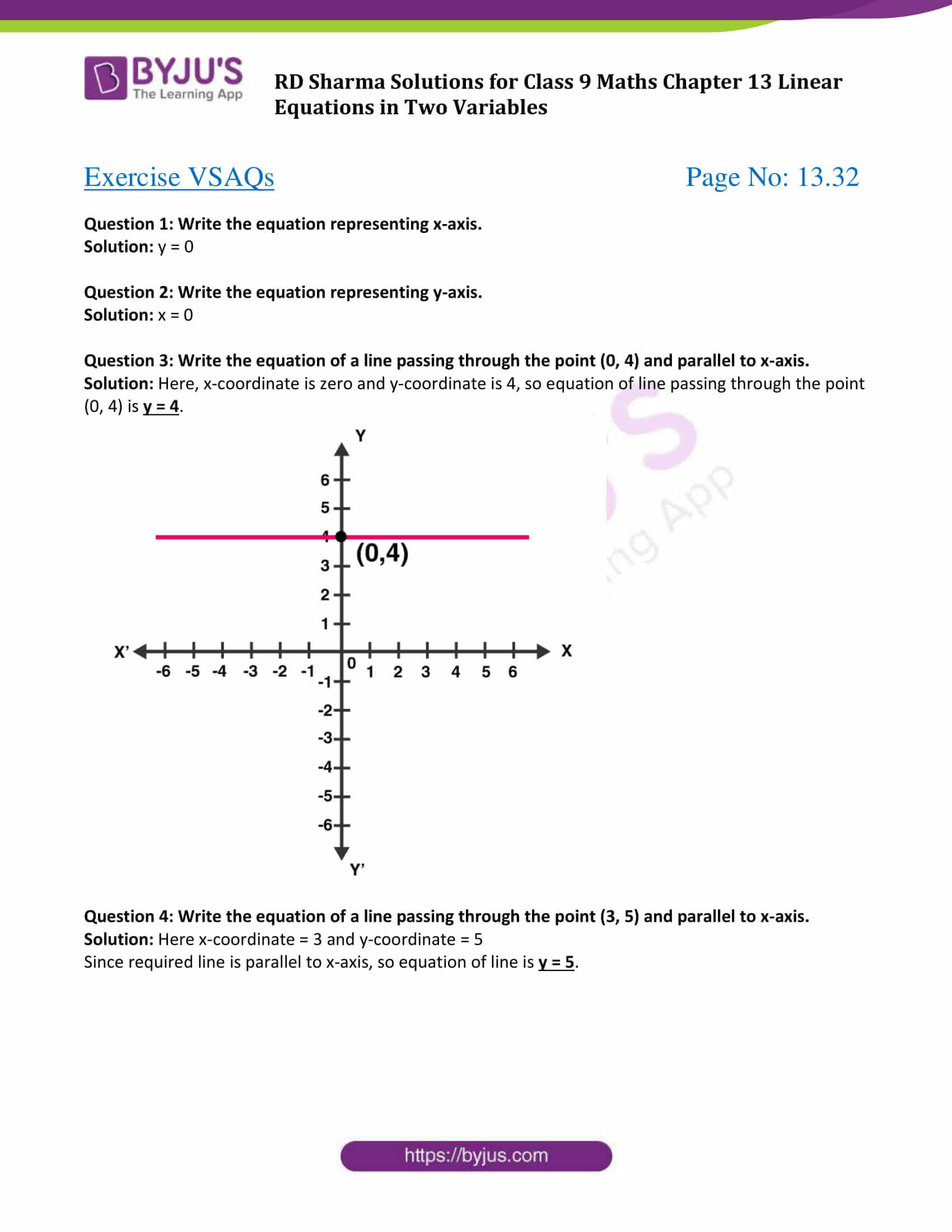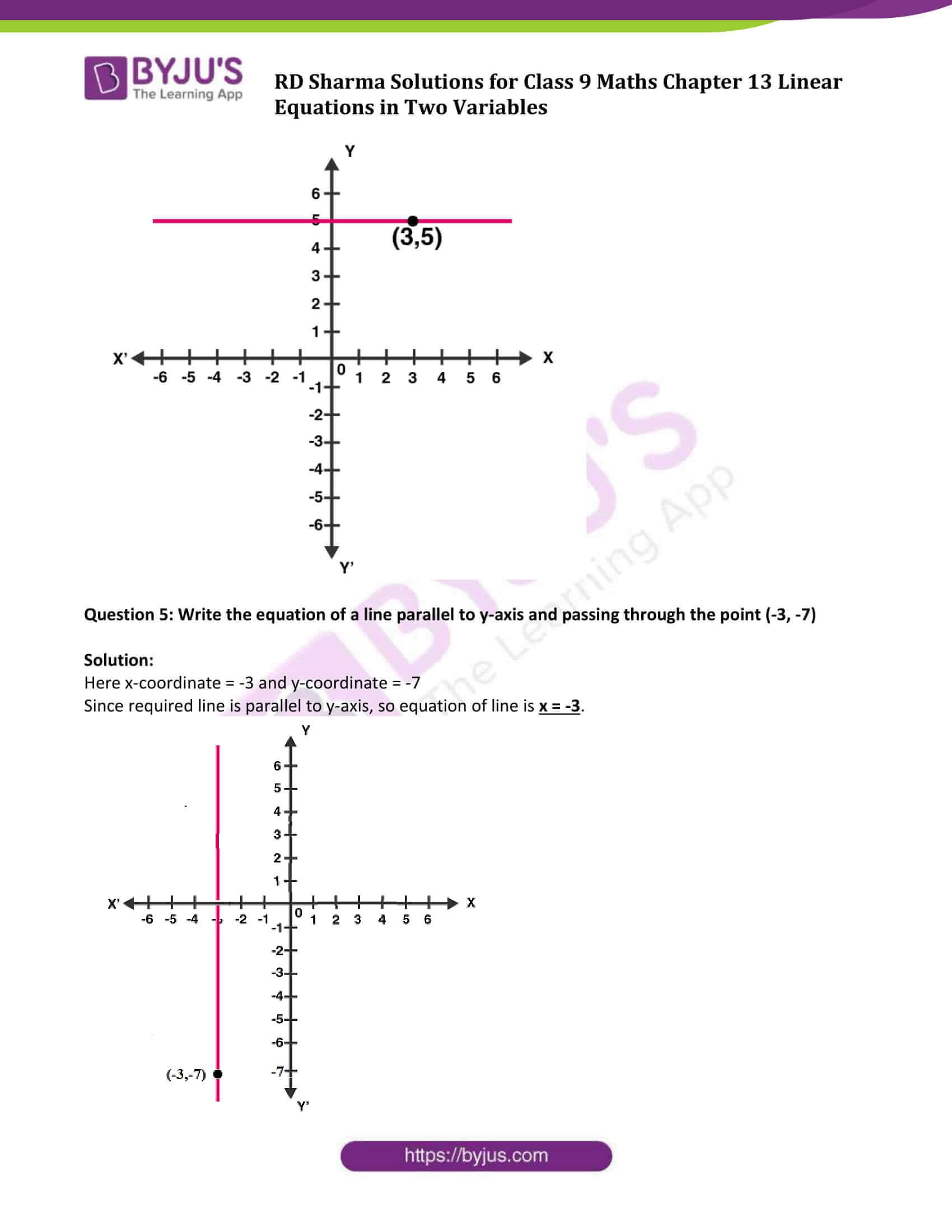### Access Answers to Maths RD Sharma Solutions for Class 9 Chapter 13 Linear Equations in Two Variables Exercise VSAQs Page number 13.32

Question 1: Write the equation representing x-axis.

Solution: y = 0

Question 2: Write the equation representing y-axis.

Solution: x = 0

Question 3: Write the equation of a line passing through the point (0, 4) and parallel to x-axis.

Solution: Here, x-coordinate is zero and y-coordinate is 4, so equation of line passing through the point (0, 4) is y = 4.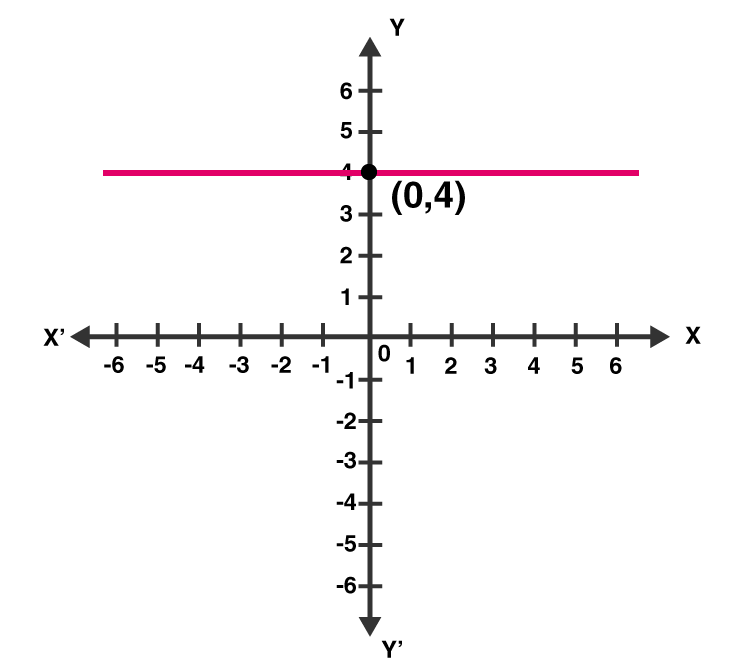Question 4: Write the equation of a line passing through the point (3, 5) and parallel to x-axis.

Solution: Here x-coordinate = 3 and y-coordinate = 5

Since required line is parallel to x-axis, so equation of line is y = 5.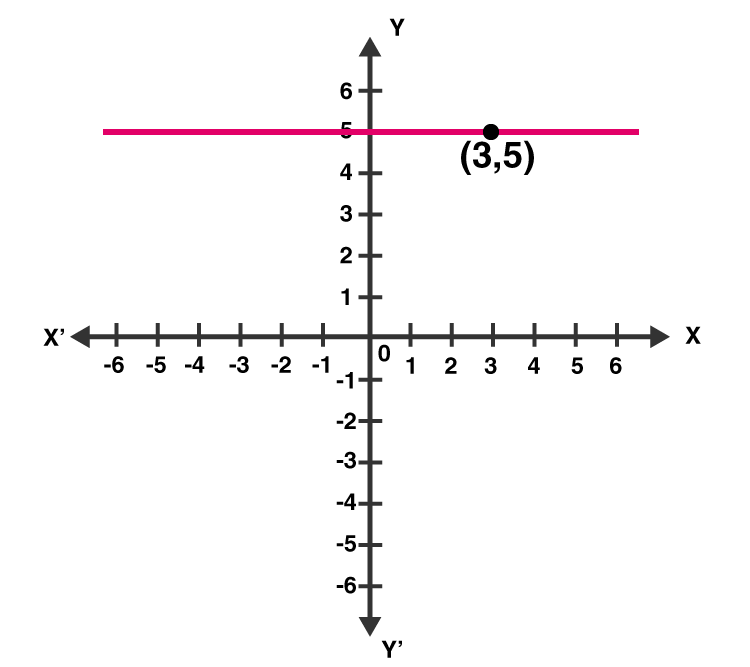Question 5: Write the equation of a line parallel to y-axis and passing through the point (-3, -7)

Solution:

Here x-coordinate = -3 and y-coordinate = -7

Since required line is parallel to y-axis, so equation of line is x = -3.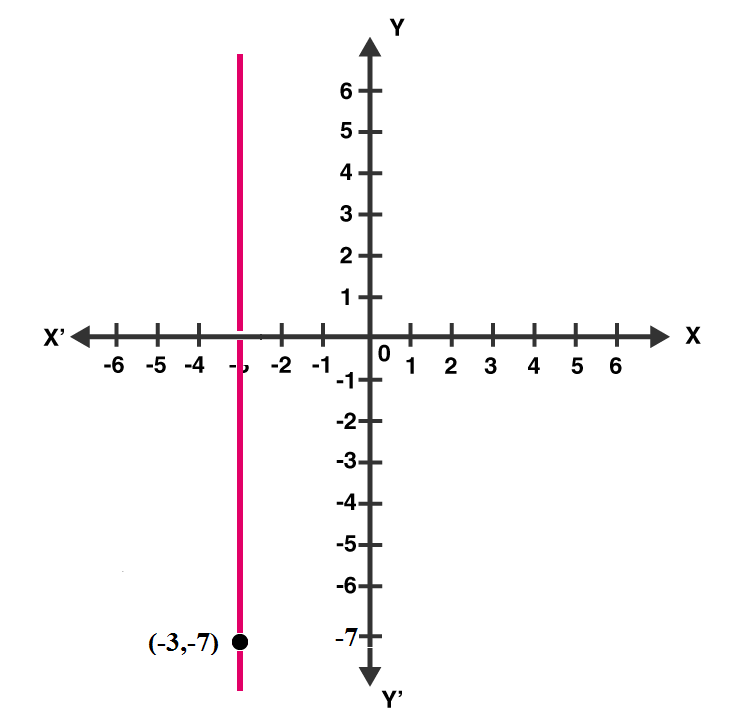## RD Sharma Solutions for Class 9 Maths Chapter 13 Linear Equations in Two Variables Exercise VSAQs

RD Sharma Solutions Class 9 Maths Chapter 13 Linear Equations in Two Variables Exercise VSAQs is based on all the concepts enlisted under the chapter. This study material helps students to understand about the linear equations and the methods to find their solutions in detail.